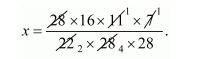# Find the depth of a cylindrical tank of radius 28 m,

Question:

Find the depth of a cylindrical tank of radius 28 m, if its capacity is equal to that of a rectangular tank of size 28 m × 16 m × 11 m.

Solution:

Let x be the depth of cylindrical tank.

The radius of tank r = 28 m.

Since,

The volume of cylindrical tank = volume of rectangular tank

$\pi r^{2} x=28 \times 16 \times 11$

$\Rightarrow \frac{22}{7} \times 28 \times 28 \times \mathrm{x}=28 \times 16 \times 11$$=\frac{16}{8}$

$x=2 \mathrm{~m}$

Thus, the depth of cylindrical tank = 2 m.